Courses

# Atoms and molecules -Important questions Class 9 Notes | EduRev

## Class 9 : Atoms and molecules -Important questions Class 9 Notes | EduRev

The document Atoms and molecules -Important questions Class 9 Notes | EduRev is a part of Class 9 category.
All you need of Class 9 at this link: Class 9

### Multiple Choice Questions

1. Which of the following correctly represents 360 g of water?
(i) 2 moles of H20
(ii) 20 moles of water
(iii) 6.022 × 1023 molecules of water
(iv) 1.2044×1025 molecules of water
• (a) (i)
• (b) (i) and (iv)
• (c) (ii) and (iii)
• (d) (ii) and (iv)
2. Which of the following statements is not true about an atom?
• (a) Atoms are not able to exist independently
• (b) Atoms are the basic units from which molecules and ions are formed
• (c) Atoms are always neutral in nature
• (d) Atoms aggregate in large numbers to form the matter that we can see, feel or touch
3. The chemical symbol for nitrogen gas is
• (a) Ni
• (b) N2
• (c) N
• (d) N
4. The chemical symbol for sodium is
• (a) So
• (b) Sd
• (c) NA
• (d) Na
5. Which of the following would weigh the highest?
• (a) 0.2 mole of sucrose (C12H22O11)
• (b) 2 moles of CO2
• (c) 2 moles of CaCO3
• (d) 10 moles of H2O
6. Which of the following has maximum number of atoms?
• (a) 18g of H2O
• (b) 18g of O2
• (c) 18g of CO2
• (d) 18g of CH4
7. Which of the following contains maximum number of molecules?
• (a) 1g CO2
• (b) 1g N2
• (c) 1g H2
• (d) 1g CH4
8. Mass of one atom of oxygen is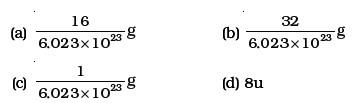9. 3.42 g of sucrose are dissolved in 18g of water in a beaker. The number of oxygen atoms in the solution are
• (a) 6.68 × 1023
• (b) 6.09 × 1022
• (c) 6.022 × 1023
• (d) 6.022 × 1021
10. A change in the physical state can be brought about
• (a) only when energy is given to the system
• (b) only when energy is taken out from the system
• (c) when energy is either given to, or taken out from the system
• (d) without any energy change

1. Which of the following represents a correct chemical formula? Name it.
• (a) CaCl
• (b) BiPO4
• (c) NaSO4
• (d) NaS
2. Write the molecular formulae for the following compounds
• (a) Copper (II) bromide
• (b) Aluminium (III) nitrate
• (c) Calcium (II) phosphate
• (d) Iron (III) sulphide
• (e) Mercury (II) chloride
• (f) Magnesium (II) acetate
3. Write the molecular formulae of all the compounds that can be formed by the combination of following ions
Cu2 , Na , Fe3 , C1, SO42-, PO43-
4. Write the cations and anions present (if any) in the following compounds
• (a) CH3COONa
• (b) NaCl
• (c) H2
• (d) NH4NO3
5. Give the formulae of the compounds formed from the following sets of elements
• (a) Calcium and fluorine
• (b) Hydrogen and sulphur
• (c) Nitrogen and hydrogen
• (d) Carbon and chlorine
• (e) Sodium and oxygen
• (f) Carbon and oxygen
6. Which of the following symbols of elements are incorrect? Give their correct symbols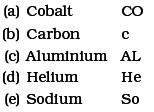7. Give the chemical formulae for the following compounds and compute the ratio by mass of the combining elements in each one of them. (You may use appendix-III).
• (a) Ammonia
• (b) Carbon monoxide
• (c) Hydrogen chloride
• (d) Aluminium fluoride
• (e) Magnesium sulphide
8. State the number of atoms present in each of the following chemical species
• (a) CO32–
• (b) PO33–
• (c) P2O5
• (d) CO
9. What is the fraction of the mass of water due to neutrons?
10. Does the solubility of a substance change with temperature? Explain with the help of an example.
11. Classify each of the following on the basis of their atomicity.
• (a) F2
• (b) NO2
• (c) N2O
• (d) C2H6
• (e) P4
• (f) H2O2
• (g) P4O10
• (H) O3
• (i) HCl
• (j) CH4
• (k) He
• (l) Ag
12. You are provided with a fine white coloured powder which is either sugar or salt. How would you identify it without tasting?
13. Calculate the number of moles of magnesium present in a magnesium ribbon weighing 12 g. Molar atomic mass of magnesium is 24g mol–1.

1. Verify by calculating that
• (a) 5 moles of CO2 and 5 moles of H2O do not have the same mass.
• (b) 240 g of calcium and 240 g magnesium elements have a mole ratio of 3:5.
2. Find the ratio by mass of the combining elements in the following compounds. (You may use Appendix-III)
• (a) CaCO3
• (b) MgCl2
• (c) H2SO4
• (d) C2H5OH
• (e) NH3
• (f) Ca(OH)2
3. Calcium chloride when dissolved in water dissociates into its ions according to the following equation.
CaCl2(aq) → Ca2 (aq) 2Cl(aq)
Calculate the number of ions obtained from CaCl2 when 222 g of it is dissolved in water.
4. The difference in the mass of 100 moles each of sodium atoms and sodium ions is 5.48002 g. Compute the mass of an electron.
5. Cinnabar (HgS) is a prominent ore of mercury. How many grams of mercury are present in 225 g of pure HgS? Molar mass of Hg and S are 200.6 g mol–1 and 32 g mol–1respectively.
6. The mass of one steel screw is 4.11g. Find the mass of one mole of these steel screws. Compare this value with the mass of the Earth (5.98 × 1024kg).
Which one of the two is heavier and by how many times?
7. A sample of vitamic C is known to contain 2.58 ×1024 oxygen atoms. How many moles of oxygen atoms are present in the sample?
8. Raunak took 5 moles of carbon atoms in a container and Krish also took 5 moles of sodium atoms in another container of same weight. (a) Whose container is heavier? (b) Whose container has more number of atoms?
9. Fill in the missing data in the Table 3.1
Table 3.1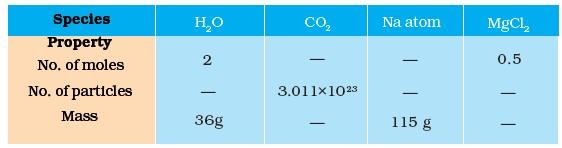10. The visible universe is estimated to contain 1022 stars. How many moles of stars are present in the visible universe?
11. What is the SI prefix for each of the following multiples and sub multiples of a unit?
• (a) 103
• (b) 10-1
• (c) 10-2
• (d) 10-6
• (e) 10-9
• (f) 10-12
12. Express each of the following in kilograms
• (a) 5.84 × 10-3mg
• (b) 58.34 g
• (c) 0.584g
• (d) 5.873 × 10-21g
13. Compute the difference in masses of 10 3 moles each of magnesium atoms and magnesium ions.
(Mass of an electron = 9.1 × 10-31kg)
14. Which has more number of atoms?
100g of N2 or 100 g of NH3
15. Compute the number of ions present in 5.85 g of sodium chloride.
16. A gold sample contains 90% of gold and the rest copper. How many atoms of gold are present in one gram of this sample of gold?
17. What are ionic and molecular compounds? Give examples.
18. Compute the difference in masses of one mole each of aluminium atoms and one mole of its ions. (Mass of an electron is 9.1 × 10–28g). Which one is heavier?
19. A silver ornament of mass ‘m’ gram is polished with gold equivalent to 1% of the mass of silver. Compute the ratio of the number of atoms of gold and silver in the ornament.
20. A sample of ethane (C2H6) gas has the same mass as 1.5 × 1020molecules of methane (CH4). How many C2H6 molecules does the sample of gas contain?
21. Fill in the blanks
• (a) In a chemical reaction, the sum of the masses of the reactants and products remains unchanged. This is called ————.
• (b) A group of atoms carrying a fixed charge on them is called ————.
• (c) The formula unit mass of Ca3(PO4)2 is ————.
• (d) Formula of sodium carbonate is ———— and that of ammonium sulphate is ————.
22. Complete the following crossword puzzle (Fig. 3.1) by using the name of the chemical elements. Use the data given in Table 3.2.
Table 3.2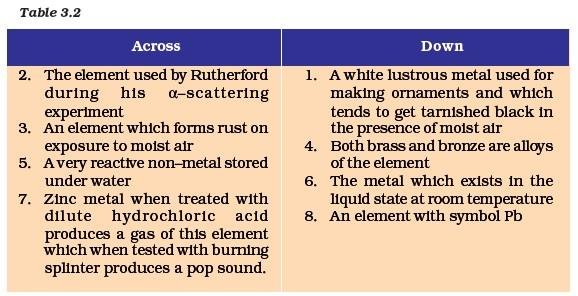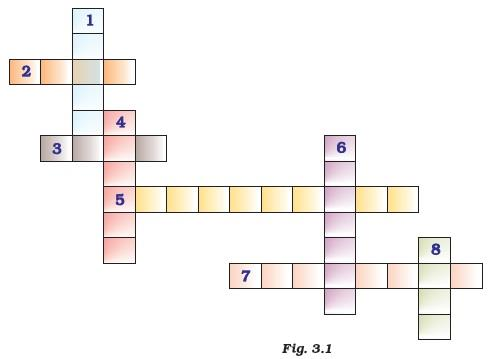23. (a) In this crossword puzzle (Fig 3.2), names of 11 elements are hidden.
Symbols of these are given below. Comple
• 2. H
• 3. Ar
• 4. O
• 5. Xe
• 6. N
• 7. He
• 8. F
• 9. Kr
• 10. Rn
• 11. Ne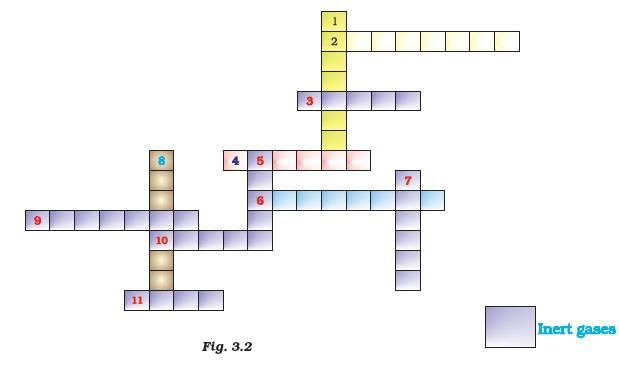• (b) Identify the total number of inert gases, their names and symbols from this cross word puzzle.
24. Write the formulae for the following and calculate the molecular mass for each one of them.
• (a) Caustic potash
• (b) Baking powder
• (c) Lime stone
• (d) Caustic soda
• (e) Ethanol
• (f) Common salt
25. In photosynthesis, 6 molecules of carbon dioxide combine with an equal number of water molecules through a complex series of reactions to give a molecule of glucose having a molecular formula C6H12O6. How many grams of water would be required to produce 18 g of glucose? Compute the volume of water so consumed assuming the density of water to be 1g cm–3
Offer running on EduRev: Apply code STAYHOME200 to get INR 200 off on our premium plan EduRev Infinity!

,

,

,

,

,

,

,

,

,

,

,

,

,

,

,

,

,

,

,

,

,

;